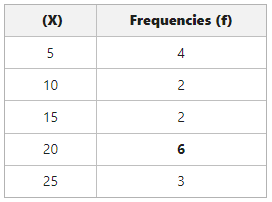# Calculation of Mode in Individual Series

• Last Updated : 26 May, 2022

The ‘word’ mode gets its roots from the old French word ‘La Mode’, which means most popular phenomenon, and from the Latin word ‘Modus’, meaning measurements, quantity, way or manner. However, in English, the literal meaning of mode is ‘the most frequent value in a set of values’. Mode, therefore, in statistics, refers to the variable that occurs most of the time in the given series. In simple words, a mode is a variable that repeats itself most frequently in a given series of variables (say X). We can determine the mode in two series; viz., individual and discrete series.

Mode is denoted as ‘Z‘.

## Individual Series :

Individual series is a series that shows the data individually. It does not involve the class or frequency of the values, which means that in individual series, each variable is shown as a single variable or separately. We can present the data either in the form of serial numbers or in ascending or descending order.

## Methods of calculating Mode in Individual Series

There are two methods of calculating Mode in Individual series:

•  Inspection Method
• Converting Individual series into discrete frequency series

### 1. Inspection Method

Inspection, in general, means observing critically. Under the Inspection method, the value of mode is simply calculated by observing the given series of variables. The number that repeats or occurs the maximum number of times is considered to be the Mode value for that series.

### Steps to calculate mode using Inspection Method in case of Individual Series

Step 1: Arrange the given series in ascending order or descending order.

Step 2: Observe which variable in the series is repeating itself and how many times.

Step 3: The third step is to determine the mode.

Now, there are four cases in which the mode of a series can be determined.

• The first one is Uni-Model. In this case, the variable that occurs the maximum number of times is considered to be the value of mode.
• The second case is Bi-Model. In this, if two variables repeat themselves an equal number of times, then both variables will be considered the value of Mode.
• The third case is Multi-Model. If in the series, more than two variables repeat themselves an equal number of times, then the value of Mode will be all such variables.
• The last case is Without Mode. When no variable in a series repeats itself, then the value of Mode is ill determined. Also, when most of the variables in a given series repeat themselves an equal number of times, then also the value of Mode is ill-determined.

#### Example 1: Calculate the mode for the following data.

1,3,5,7,5,9,5,11

Solution:

Step 1: Arrange the given series in ascending order

1,3,5,5,5,7,9,11

Step 2: Observe

Only variable 5 repeats itself three times.

Mode (z)=5 [Uni-Model]

#### Example 2: Calculate the mode for the following data.

2,2,4,6,8,10,12,12,14,16

Solution:

Step 1: Arrange the given series in ascending order

2,2,4,6,8,10,12,12,14,16

Step 2: Observe

Variable 2 and 12 occur twice in the series.

Mode (Z)= 2 and 12 [Bi-Model]

#### Example 3: Calculate the mode for the following data.

2,4,6,12,8,10,12,14,16,22,16,26,25,26

Solution:

Step 1: Arrange the given series in ascending order

2,4,6,8,10,12,12,14,16,16,22,25,26,26

Step 2: Observe

Variable 12,16 and 26 occur twice in the series.

Mode (Z)= 12,16 and 26 [Multi-Model]

#### Example 4: Calculate the mode for the following data.

1,2,3,4,5,6

Solution:

Step 1: Arrange the given series in ascending order

1,2,3,4,5,6

Step 2: Observation

No variable in the series repeats itself.

Mode (Z) = ill-determined

### 2. Converting Individual Series into Discrete Frequency Series

When the number of values in an individual series is large, it becomes difficult to determine the mode through the inspection method. In such cases, we use the second method, where firstly, individual series is converted into discrete series, and then the mode is determined using the inspection method unless otherwise mentioned.

#### Steps to Calculate Mode using Inspection Method in case of Conversion of individual series into discrete frequency series

Step 1: Arrange the given series in ascending order.

Step 2:  Prepare a table with two columns, Column 1 showing variable (X) and column 2 showing the frequency of each variable, respectively, against that variable.

Step 3: Use the Inspection method to determine the mode of the given series. The variable with the highest frequency will be the mode.

#### Example: Calculate the mode for the following data.

5,10,5,10,5,15,5,15,20,25,20,25,20,20,20,25,20.

Solution:

Step 1: Arrange the given series in ascending order.

5,5,5,5,10,10,15,15,20,20,20,20,20,20,25,25,25.

Step 2: Prepare a table with two columns. Column 1 shows variables (X), and column 2 shows the frequency (number of times it occurs in the series) of each variable, respectively, against that variable.Step 3: Use the Inspection method to determine the Mode of the given series.

The variable 20 has the highest frequency value of 6. So, the Mode (Z) = 20.

My Personal Notes arrow_drop_up
Recommended Articles
Page :